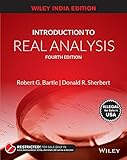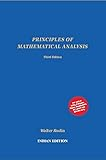# CSIR-NET: Analysis/Topology Quiz 3

For 𝛼∈ℝ let ⌊𝛼⌋ denote the greatest integer smaller than or equal to 𝛼. Define 𝑑:ℝ×ℝ→[0,∞) by 𝑑(𝑥,𝑦)=⌊|𝑥−𝑦|⌋, 𝑥,𝑦∈ℝ. Then which of the following are true?

d(x,y)=0, if and only if x=y, x,y∈ℝ
What can you say about d(0.2,0.4)?

d(x,y)=d(y,x), x,y∈ℝ
Clearly, since |x – y| = |y – x|, we can be sure that this option is true.

d(x,y) ≤ d(x,z) + d(z,y), x,y,z∈ℝ
What happens if x = 1.2, y = 2.2, z = 2?

d is not a metric on ℝ
Verifying option 3, triangular inequality is not satisfied

CSIR-NET December 2019, Part C Question.

Do you have any other ways to prove/disprove options above?

Beauty of the entire question is that you simply need to understand the definition of metric spaces well to find the answer. Watch the video below to learn more.

## Recommended Books for Real AnalysisIntroduction to Real Analysis by Bartle and Sherbert (4e)Principles of Mathematical Analysis by Rudin